Courses

# NCERT Exemplar (Part - 2) - Solid State Notes | EduRev

## Class 12 : NCERT Exemplar (Part - 2) - Solid State Notes | EduRev

The document NCERT Exemplar (Part - 2) - Solid State Notes | EduRev is a part of the Class 12 Course Chemistry Class 12.
All you need of Class 12 at this link: Class 12

Q.54. Why are liquids and gases categorised as fluids?
Ans. The liquids and gases have a property to flow i.e., the molecules of liquids and gases can easily move fast and tumble over one another freely. Because of their tendency to flow, these have been categorized as fluids.

Q.55. Why are solids incompressible?
Ans. The intemuclear distance between the constituent particles (atoms, molecules or ions) in solids are very less. On bringing them further closer, there will be large repulsive force between electron clouds of these particles. Therefore, solids cannot be compressed.

Q.56. Inspite of long range order in the arrangement of particles why are the crystals usually not perfect?
Ans. Crystals have long range in the arrangement of particles but usually the crystals are not perfect this is because when crystallisation occurs at a fast rate or moderate rate, the constituent particles may not get sufficient time to arrange themselves in a perfect order.

Q.57. Why does table salt, NaCl, some times appear yellow in colour?
Ans. The yellow colour of sodium chloride crystals is due to metal excess defect. In this defect, the unpaired electrons get trapped in anion vacancies. These sites are called F-centres. The‘yellow colour results by excitation of these electrons when they absorb energy from the visible light falling on the crystals.

Q.58. Why is FeO(s) not formed in stoichiometric composition?
Ans. Iron oxide (FeO) has rock salt structure.
In this case, O2 ions adopt on sites and Fe2+ ions should occupy non- stoichiometric. This is the ideal arrangement.
This oxide is always non-stoichiometric i.e., the composition of Fe2+ and O2 ions is not 1 : 1. It is 0.95 : 1
i.e. Fe0.95(Wustite)
This composition can be obtained if a small number of Fe2+ ions are replaced by two-thirds of Fe3+ ions in Oh sites.
Eventually there would be less amount of metal as compared to stoichiometric composition.

Q.59. Why does white ZnO(s) becomes yellow upon heating?
Ans. When ZnO is heated, it splits up to give Zn2+, electrons and colour because of the following reasons:
The excess Zn ions thus formed get entrapped in the interstitial site and electron in the neighborhood vacant interstitial sites. This electron is responsible for the colour and electrical conductivity in crystals.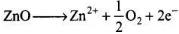• ZnO heated in Zn vapour — Zny0 (y > I) • The excess cations occupy interstitial voids • The electrons (2e-) released stay associated to the interstitial cation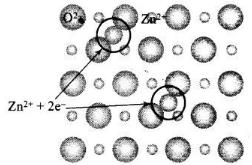Q.60. Why does the electrical conductivity of semiconductors increase with rise in temperature?
Ans. The energy gap between valence band and conduction band is small. At room temperature, they do not conduct electricity but when temperature is raised large number of electron from valence band get sufficient energy to jump to conduction band. This is known as thermodynamic conduction in intrinsic semiconductors. Thus, they become more conducting as the temperature increases.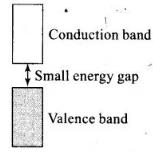Q.61. Explain why does conductivity of germanium crystals increase on doping with gallium.
Ans. p-type semiconductor: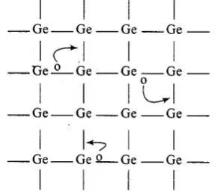• When Ge is doped with group 13 elements, for example, gallium, the structure of crystal lattice does not change.
• 3 valence electrons of gallium are used up in the normal covalent bond.
• For one dopant atom, one hole is created because the place where fourth electron is missing is called vacancy or hole and is responsible for conduction of germanium doped with gallium.

Electron from neighbouring atom comes and fills the hole, thereby creating a hole in its original position.Under the influence of electric field electrons move towards positively charged plates through these and conduct electricity. The holes appear to move towards negatively charged plates.

Q.62. In a compound, nitrogen atoms (N) make cubic close packed lattice and metal atoms (M) occupy one-third of the tetrahedral voids present. Determine the formula of the compound formed by M and N?
Ans. In ccp, no. of atoms per unit cell = 4
Thus, of tetrahedral voids = 2 x No. of atoms in ccp = 2 x 4 = 8
Only one-third of tetrahedral voids are occupied by metal M. No. of
No. of metal atoms, M = 1/ x 8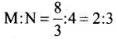Thus, formula is M2N3.

Q.63. Under which situations can an amorphous substance change to crystalline form?
Ans. An amorphous solid on heating at some temperature may become crystalline. Slow heating and cooling over a long period makes an amorphous solid acquires some crystalline character.

MATCHING TYPE

Note : In the following questions match the items given in Column I with the items given in Column II. In some questions more than one item of Column I and Column II may match.
Q.64. Match the defects given in Column I with the statements in given Column II.

 Column I Column II (i) Simple vacancy defect (a) shown by non-ionic solids and increases density of the solid. (ii) Simple interstitial defect (b) shown by ionic solids and decreases density of the solid. (iii) Frenkel defect (c) shown by non ionic solids and density of the solid decreases (iv) Schottky defect (d) shown by ionic solids and density of the solid remains the same.

Ans. (i) → (c); (ii) → (a); (iii) → (d); (iv) → (b)
Solution.
(i) When some of lattice sites are vacant in any non-ionic solid, the crystal is said to have vacancy defect and due to decrease in number of particles present in crystal lattice the density of crystal decreases.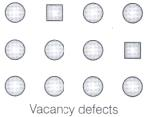(ii) Simple interstitial defect are shown by non-ionic solids in which constituent particles is displaced from its normal site to an interstitial site. Hence, density of solid increases.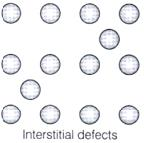(iii) Frenkel defect is shown by ionic solids in which smaller ions get dislocated from its normal site to its interstitial site which lead to decrease its density.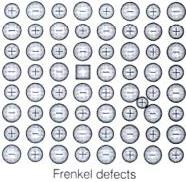(iv) Schottky defect is shown by ionic solids in which equal number of cation and anion get missed from ionic solids and thus, density of solid decreases.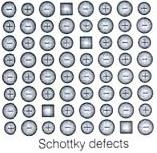Q.65. Match the type of unit cell given in Column I with the features given in Column II.

 Column I Column II (i) Primitive cubic unit cell (a) Each of the three perpendicular edges compulsorily have the different edge length i.e;  a≠b≠c. (ii) Body centred cubic unit cell (b) Number of atoms per unit cell is one. (iii) Face centred cubic unit cell (c) Each of the three perpendicular edges compulsorily have the same edge length i.e; a = b = c (iv) End centred orthorhombic unit cell (d) In addition to the contribution from the corner atoms the number of atoms present in a unit cell is one. (e) In addition to the contribution from the corner atoms the number of atoms present in a unit cell is three.

Ans. (i) → (b, c); (ii) → (c, d); (iii) → (c, e); (iv) → (a, d)
Solution.
(i) For primitive unit cell, a = b = c
Total number of atoms per unit cell = 1 / 8 x 8 = 1
Here, 1/8 is due to contribution of each atom present at corner.
(ii) For body centered cubic unit cell, a = b = c
This lattice contains atoms at corner as well as body centre. Contribution due to atoms at corner = 1 / 8 x 8 = 1 contribution due to atoms at body centre = 8
(iii) For face centred unit cell, a = b = c
Total constituent ions per unit cell present at corners = 1 / 8 x 8 = 1
Total constituent ions per unit cell present at face centre = 1 / 2 x 6 = 3
(iv) For end centered orthorhombic unit cell, a ≠ b ≠ c
Total contribution of atoms present at corner = 1 / 8 x 8 = 1
Total contribution of atoms present at end centre = 1 / 2 x 2 = 1
Hence, other than corner it contains total one atom per unit cell.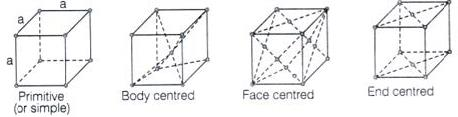Q.66. Match the types of defect given in Column I with the statement given in Column II.

 Column I Column II (i) Impurity defect (a) NaCl with anionic sites called F-centres (ii) Metal excess defect (b) FeO with Fe3+ (iii) Metal deficiency defect (c) NaCl with Sr2+ and some cationic sites vacant

Ans. (i) → (c); (ii) → (a); (iii)→ (b)
Solution.
(i) Impurity defects: The defects introduced in the crystal lattice due to presence of the certain impurity are called impurity defects.
Example: Substitution of Na+ ions in NaCl by Sr2+ ions.
Structure with defect: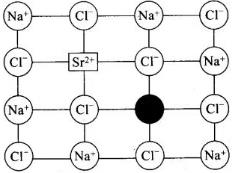Impurity defect due to substitution of Naions in NaCl by Sr2+ ions (Cation vacancy) ‘Schottky Defect’
(ii) When NaCl is heated in vapour of sodium some of the Cf leave their lattice site and create anion vacancies. This chloride ion wants to combine with sodium vapour to form sodium chloride. For doing so sodium atom loses electrons form Na+ ions. This released electron diffuses into the crystal to get entrapped in the anion vacancy called F-centre.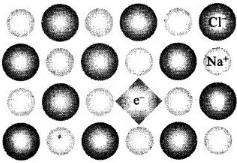(iii) Metal deficiency is caused due to cation vacancy created by replacement of some lower valent ions by its higher valentions.
Note: Cation vacancies are found in crystals in which metals have different oxidation states.
Example: FeO, FeS, NiO

Q.67. Match the items given in Column I with the items given in Column II.

 Column I Column II (i) Mg in solid state (a) p-Type semiconductor (ii) MgCl2 in molten state (b) n-Type semiconductor (iii) Silicon with phosphorus (c) Electrolytic conductors (iv) Germanium with boron (d) Electronic conductors

Ans. (i) → (d); (ii) → (c); (iii) → (b); (iv) →(a)
Solution.
(i) Mg in solid state show electronic conductivity due to presence of free electrons hence, they are known as electronic conductors.
(ii) MgCl2 in molten state show electrolytic conductivity due to presence of electrolytes in molten state.
(iii) Silicon doped with phosphorus contain one extra electron due to which it shows conductivity under the influence of electric field and known as p-type semiconductor.
(iv) Germanium doped with boron contains one hole due to which it shows conductivity under the influence of electric field and known as n-type semiconductor.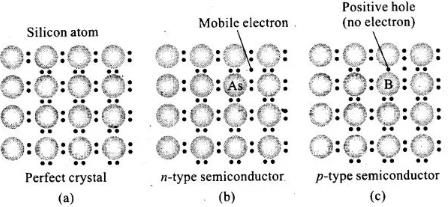Q.68. Match the type of packing given in Column I with the items given in Column II.

 Column I Column II (i) Square close packing in two dimensions (a) Triangular voids (ii) Hexagonal close packing in two dimensions (b) Pattern of spheres is repeated in every fourth layer (iii) Hexagonal close packing in three dimensions (c) Coordination number = 4 (iv) Cubic close packing in three dimensions (d) Pattern of sphere is repeated in alternate layers

Ans. (i) → (c); (ii) → (a); (iii) → (d); (iv) → (b)
Solution.
(i) Square close packing in two dimensions each sphere have coordination number 4, as shown below.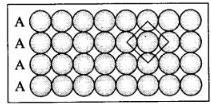(ii) Hexagonal close packing in two dimensions each sphere has coordination number 6 as shown below and creates a triangular void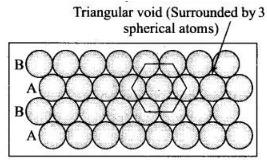(iii) Hexagonal close packing in 3 dimensions is a repeated pattern of sphere in alternate layers also known as ABAB pattern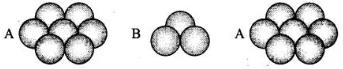(iv) Cubic close packing in a 3 dimensions is a repeating pattern of sphere in every fourth layer.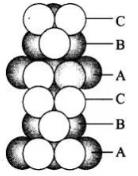ASSERTION AND REASON TYPE

Note : In the following questions a statement of assertion followed by a statement of reason is given.
Choose the correct answer out of the following choices.
(i) Assertion and reason both are correct statements and reason is correct explanation for assertion.
(ii) Assertion and reason both are correct statements but reason is not correct explanation for assertion.
(iii) Assertion is correct statement but reason is wrong statement.
(iv) Assertion is wrong statement but reason is correct statement.

Q.69. Assertion: The total number of atoms present in a simple cubic unit cell is one.
Reason: Simple cubic unit cell has atoms at its corners, each of which is shared between eight adjacent unit cells.
Ans. (i)
Solution.
In simple cubic unit cell, only comers are occupied by atoms. Thus, total number of atoms present in the unit cell will be one.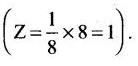Q.70. Assertion: Graphite is a good conductor of electricity however diamond belongs to the category of insulators.
Reason: Graphite is soft in nature on the other hand diamond is very hard and brittle.
Choose the correct answer out of the following choices.
(i) Assertion and reason both are correct statements and reason is correct explanation for assertion.
(ii) Assertion and reason both are correct statements but reason is not correct explanation for assertion.
(iii) Assertion is correct statement but reason is wrong statement.
(iv) Assertion is wrong statement but reason is correct statement.

Ans. (ii)
Solution.
Diamond is bad conductor of electricity because all valence of carbon are involved in bonding. In graphite however 3 out of 4 valence electrons are involved in bonding, fourth electron remains free between adjacent layers which makes it a good conductor.
Graphite is soft because parallel layers are held together by week van der Waals force. However, diamond is hard due to compact three-dimensional network of bonding.

Q.71. Assertion: Total number of octahedral voids present in unit cell of cubic close packing including the one that is present at the body centre, is four.
Reason: Besides the body centre there is one octahedral void present at the centre of each of the six faces of the unit cell and each of which is shared between two adjacent unit cells.
Choose the correct answer out of the following choices.
(i) Assertion and reason both are correct statements and reason is correct explanation for assertion.
(ii) Assertion and reason both are correct statements but reason is not correct explanation for assertion.
(iii) Assertion is correct statement but reason is wrong statement.
(iv) Assertion is wrong statement but reason is correct statement.

Ans. (iii)
Solution.
All edge centres and body centre represent octahedral void.
Total number of octahedral voids = 12 x 1/4 +1 = 4

Q.72. Assertion : The packing efficiency is maximum for the fcc structure.
Reason: The cordination number is 12 in fcc structures.
Choose the correct answer out of the following choices.
(i) Assertion and reason both are correct statements and reason is correct explanation for assertion.
(ii) Assertion and reason both are correct statements but reason is not correct explanation for assertion.
(iii) Assertion is correct statement but reason is wrong statement.
(iv) Assertion is wrong statement but reason is correct statement.

Ans. (ii)
Solution.
In fcc unit cell, there is cep arrangement with packing efficiency of 74.01% which is maximum. In cep arrangement, coordination number is 12.

Q.73. Assertion: Semiconductors are solids with conductivities in the intermediate range from 10–6 – 104 ohm–1m–1.
Reason: Intermediate conductivity in semiconductor is due to partially filled valence band.
Choose the correct answer out of the following choices.
(i) Assertion and reason both are correct statements and reason is correct explanation for assertion.
(ii) Assertion and reason both are correct statements but reason is not correct explanation for assertion.
(iii) Assertion is correct statement but reason is wrong statement.
(iv) Assertion is wrong statement but reason is correct statement.

Ans. (iii)
Solution.
Conductance of semiconductors lies between metals and insulators, i.e., in the range of 10-6 - 10ohm -1 m-1 .

LONG ASWER TYPE QUESTIONS

Q.74. With the help of a labelled diagram show that there are four octahedral voids per unit cell in a cubic close packed structure.
Ans. Cubic close packed structure contains one atom at each of eight corners of a unit cell and one atom at each of six faces which can be represented below:
As we know that any atom surrounded by six atoms (hard sphere) creates an octahedral void. In case of fcc body centre is surrounded by six identical atoms present at face centre hence, there is a octahedral void at body centre of each unit cell.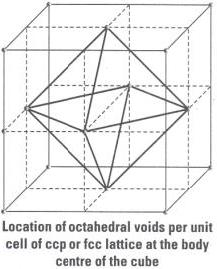Beside the body centre there is one octahedral void at centre of each of 12 edge as shown below: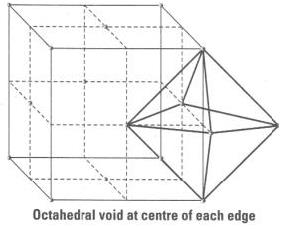Since, each void is shared by 4 unit cell. Therefore, contribution of octahedral void to each edge of a unit cell is 1/4.
Number of octahedral void at centre of 12 edge = 1 / 4 x 12 = 3
Number of octahedral void at body centre = 1
Therefore, total number of octahedral void at each ccp lattice = 3 + 1 = 4

Q.75. Show that in a cubic close packed structure, eight tetrahedral voids are present per unit cell.
Ans. Cubic close packed structure contains one atom at each corner of an unit cell and at face centre of each unit cell. Each unit cell consists of 8 small cubes.
Each small cube contains 4 atoms at its alternate corner when these atoms are joined to each other lead to creation of a tetrahedral void as shown below: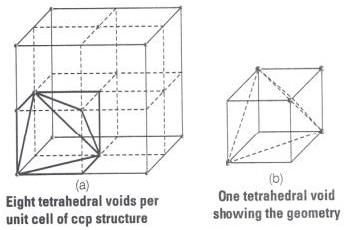Since, there are total 8 smaller cubes present at one unit cell and each smaller cube has one tetrahedral void hence, total number of tetrahedral void present in each unit cell is equal to eight.
As we know ccp structure has 4 atoms per unit cell. Thus, total number of tetrahedral void in one ccp unit cell is equal to 8.

Q.76. How does the doping increase the conductivity of semiconductors?
Ans. The conductivity of semiconductors is increased by adding an appropriate amount of suitable impurity or doping. Doping can be done with an impurity which is electron rich or electron deficient as compared to the intrinsic semiconductor, silicon or germanium. Such impurities introduce electronic defects in them. When silicon is doped with electron rich impurities the extra electron becomes delocalized. These delocalized electrons increase the conductivity of doped silicon due to the negatively charged electron, hence silicon doped with electron-rich impurity is called n-type semiconductor while electron-deficit impurities increase the conductivity through positive holes and this type of semiconductors are called /?-type semiconductors.

Q.77. A sample of ferrous oxide has actual formula Fe0.93O1.00. In this sample what fraction of metal ions are Fe2+ ions? What type of non-stoichiometric defect is present in this sample?
Ans. Let the formula of the sample be (Fe2+)x (Fe3+ )y O
On looking at the given formula of the compound
x + y = 0.93 …..(i)
Total positive charge on ferrous and ferric ions should balance the two units of negative charge on oxygen
Therefore, 2x + 3y = 2 ......(ii)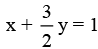.......(iii)
On subtracting equation (i) from equation (iii) we have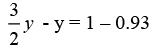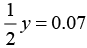⇒ y = 0.14
On putting the value of y in equation (i), we get
x + 0.14 = 0.93
⇒ x = 0.93 - 0.14
⇒ x = 0.79
Fraction of Fe2+ ions present in the sample =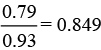Metal deficiency defect is present in the sample because iron is less in amount than that required for stoichiometric composition.

Offer running on EduRev: Apply code STAYHOME200 to get INR 200 off on our premium plan EduRev Infinity!

,

,

,

,

,

,

,

,

,

,

,

,

,

,

,

,

,

,

,

,

,

;